### Home > CALC > Chapter Ch3 > Lesson 3.1.1 > Problem3-18

3-18.
1. Evaluate each limit. If the limit does not exist, say so but also state if y is approaching positive or negative infinity. Homework Help ✎

1.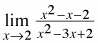2.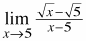3.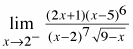4.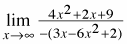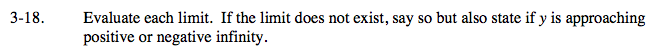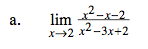Factor the numerator and denominator. If you can cancel out an (x−2), then the limit exists. If not, then it DNE.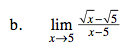You could factor the denominator. Or you could multiply the top and bottom by the conjugate of

$(\sqrt{x}-5)$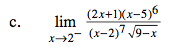The (x−2) in the denominator will not cancel out. This means that there is a vertical asymptote at x = 2. How can we determine if the asymptote approaches +∞ or −∞ as x → 2 from the left?

Choose a value that is to left of x = 2, and evaluate. Note: we only need to determine if each term is positive or negative. Choose x → 1.9:

$\lim_{x\rightarrow 1.9}\frac{(2x+1)(x-5)^{6}}{(x-2)^{7}\sqrt{9-x}}=\lim_{x\rightarrow 1.9}\frac{(+)(-)^{6}}{(-)^{7}(\sqrt{+})}$

$=\lim_{x\rightarrow 1.9}\frac{(+)(+)}{(-)(+)}= -\text{ value}$

DNE but y → −∞.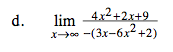This is a limit x→∞. That means we are looking at end behavior. Is there a horizontal asymptote, or do the y-values approach ∞ or −∞?

Consider only the terms with the highest power in the numerator and the denominator. Also consider their coefficients, and their signs.